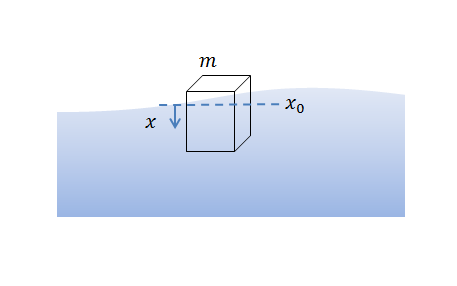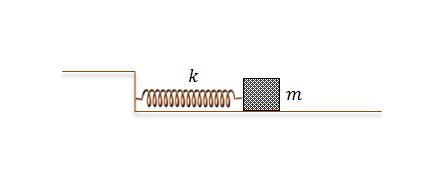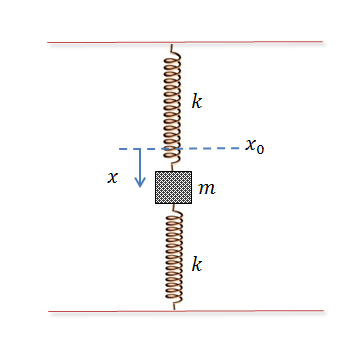Classical Mechanics

# Solutions to Simple Harmonic MotionA $40\text{ g}$ cube of edge length $l=3\text{ cm}$ floats on water, oscillating up and down. Initially at $t=0,$ the position of the body was $x(t=0) = 5 \text{ cm }$ and the velocity was $v(t=0) = 0 \text{ m/s },$ where $x$ denotes the vertical difference in the position of the cube from the equilibrium point $x_0.$ What is the velocity $v(t)$ of the body at $t = 2 \text{ s } ?$

Assumptions and Details

• Positive sign of $x(t)$ means downward direction.
• Ignore any friction.
• The density of water is $\rho = 1 \text{ g/cm}^3.$
• The gravitational acceleration is $g=10\text{ m/s}^2.$As illustrated in the above diagram, a body of mass $m = 1 \text{ kg}$ attached to a spring with spring constant $k = 9 \text{ N/m}$ is oscillating on a frictionless floor. Initially at $t=0,$ the position of the body was $x(t=0) = 1 \text{ m }$ and the velocity was $v(t=0) = 0 \text{ m/s },$ where $x$ denotes the difference in the length of the spring from its original length.

What is the position of the body at $t = 3 \text{ s } ?$A $40\text{ g}$ cube of edge length $l=5\text{ cm}$ floats on water, oscillating up and down. Initially at $t=0,$ the position of the body was $x(t=0) = 4 \text{ cm }$ and the velocity was $v(t=0) = 0 \text{ m/s },$ where $x$ denotes the vertical difference in the position of the cube from the equilibrium point $x_0.$ What is $x(t)$ at $t = 2 \text{ s } ?$

Positive sign of $x(t)$ means downward direction.
Ignore any friction.
The density of water is $\rho = 1 \text{ g/cm}^3.$
The gravitational acceleration is $g=10\text{ m/s}^2.$As illustrated in the above diagram, a body of mass $m = 3 \text{ kg}$ attached to a spring with spring constant $k = 75 \text{ N/m}$ is oscillating on a frictionless floor. Initially at $t=0,$ the position of the body was $x(t=0) = 1 \text{ m }$ and the velocity was $v(t=0) = 0 \text{ m/s },$ where $x$ denotes the difference in the length of the spring from its original length. What is the velocity of the body at $t = 5 \text{ s } ?$A body of mass $m = 6 \text{ kg}$ is oscillating on two identical springs with spring constant $k = 48 \text{ N/m},$ as shown in the above diagram, where $x$ denotes the downward difference from the equilibrium point $x_0.$ Initially, the difference of the body was $x(t=0) = 4 \text{ m }$ and the velocity was $v(t=0) = 0 \text{ m/s }.$ What is the position of the body at $t = 4 \text{ s } ?$

Assumptions and Details

• The gravitational acceleration is $g = 10 \text{m/s}^2.$
×